# 精准营销！用机器学习完成客户分群！# 💡 核心步骤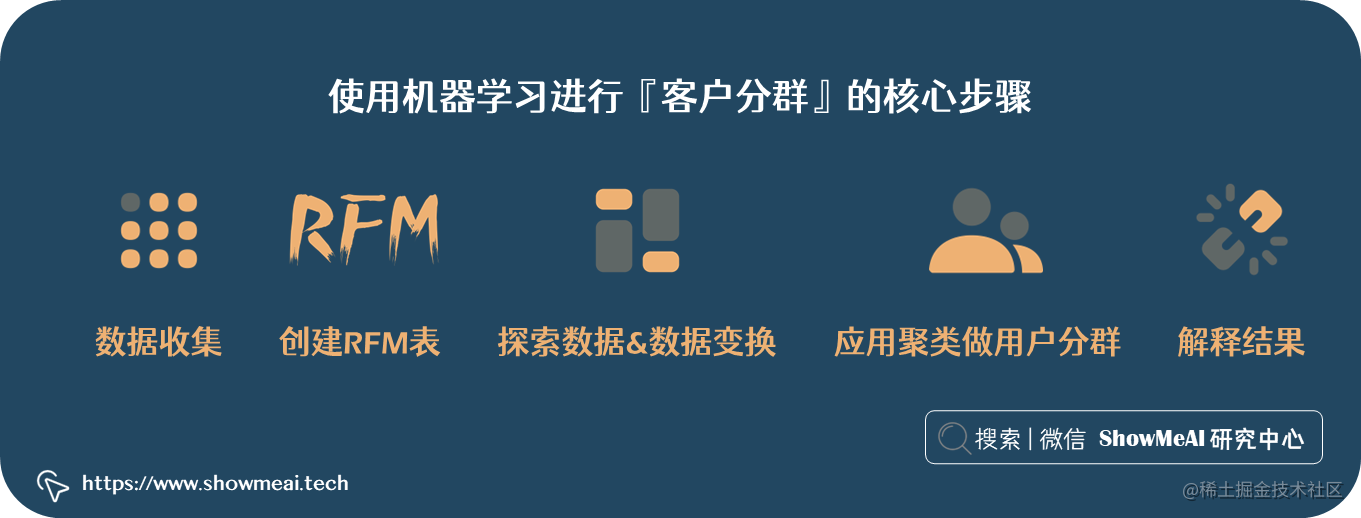• 数据收集
• 创建RFM表
• 探索数据&数据变换
• 应用聚类做用户分群
• 解释结果

# 💡 数据收集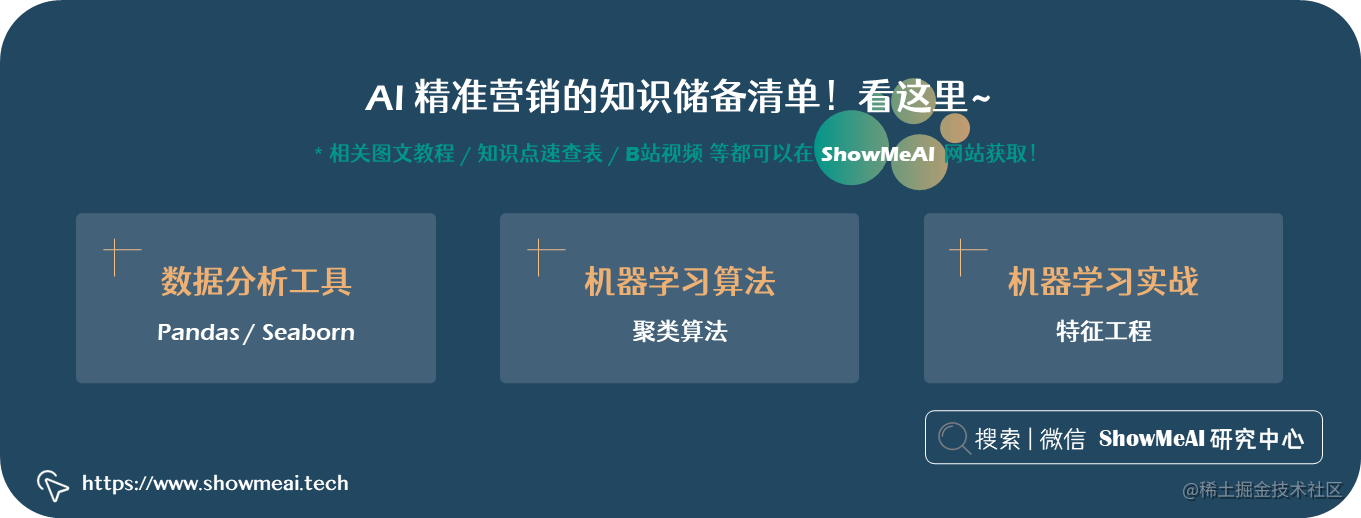🏆 实战数据集下载（百度网盘）：公众号『ShowMeAI研究中心』回复『实战』，或者点击 这里 获取本文 基于机器学习的用户价值数据挖掘与客户分群Online_Retail 在线零售数据集

ShowMeAI官方GitHubgithub.com/ShowMeAI-Hu…

``````# 导入工具库
import pandas as pd
import matplotlib.pyplot as plt
import numpy as np

# 读取数据
df = df[df['CustomerID'].notna()]

# 数据采样
df_fix = df.sample(10000, random_state = 42)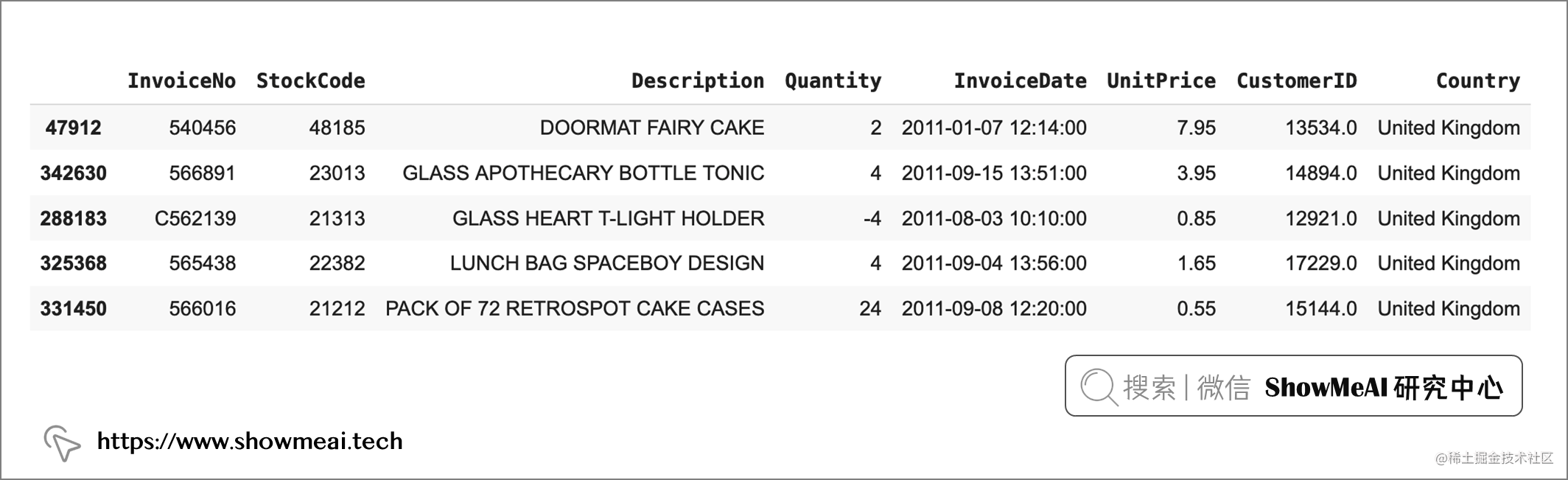# 💡 创建 RFM 表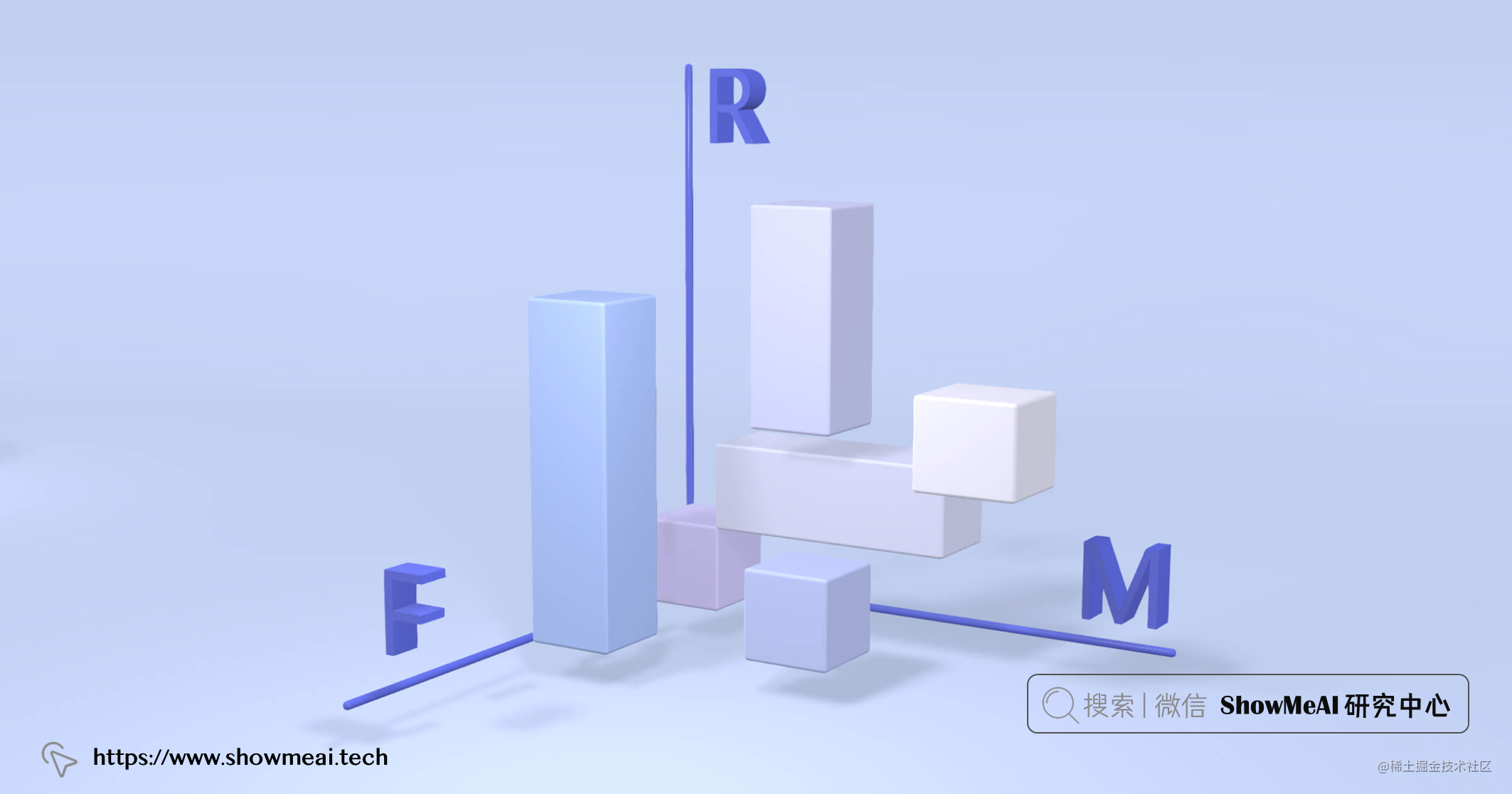• 可以用事务发生的日期减去快照日期，来代表最近消费时间点。
• 可以计算每个客户的交易量，作为频度信息。
• 可以汇总每个客户的所有交易金额，作为消费金额列。

``````# 只保留日期
from datetime import datetime
df_fix["InvoiceDate"] = df_fix["InvoiceDate"].dt.date

# 总金额
df_fix["TotalSum"] = df_fix["Quantity"] * df_fix["UnitPrice"]

# 最近消费时间点快照
import datetime
snapshot_date = max(df_fix.InvoiceDate) + datetime.timedelta(days=1)

# 统计聚合
customers = df_fix.groupby(['CustomerID']).agg({
'InvoiceDate': lambda x: (snapshot_date - x.max()).days,
'InvoiceNo': 'count',
'TotalSum': 'sum'})

# 重命名字段
customers.rename(columns = {'InvoiceDate': 'Recency',
'InvoiceNo': 'Frequency',
'TotalSum': 'MonetaryValue'}, inplace=True)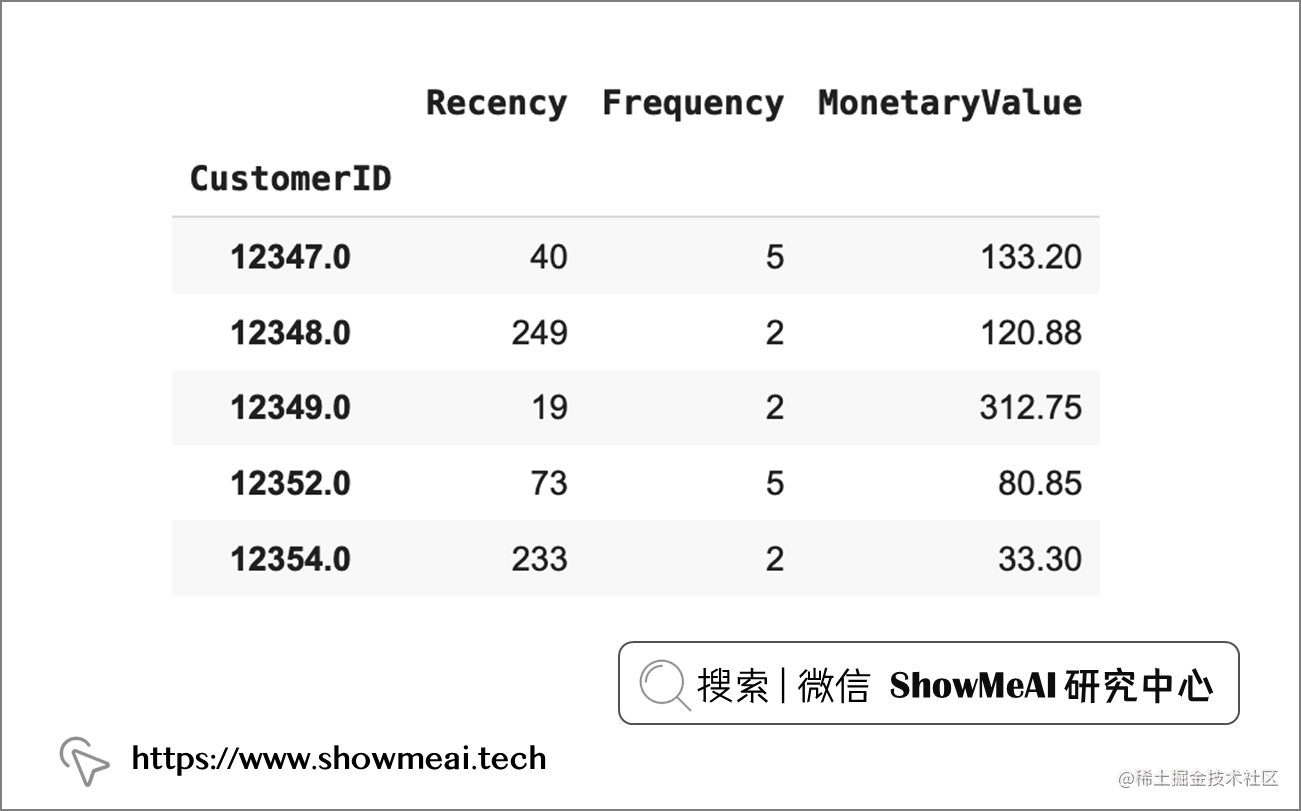# 💡 探索数据&数据变换

``````fig, ax = plt.subplots(1, 3, figsize=(15,3))
sns.distplot(customers['Recency'], ax=ax)
sns.distplot(customers['Frequency'], ax=ax)
sns.distplot(customers['MonetaryValue'], ax=ax)
plt.tight_layout()
plt.show()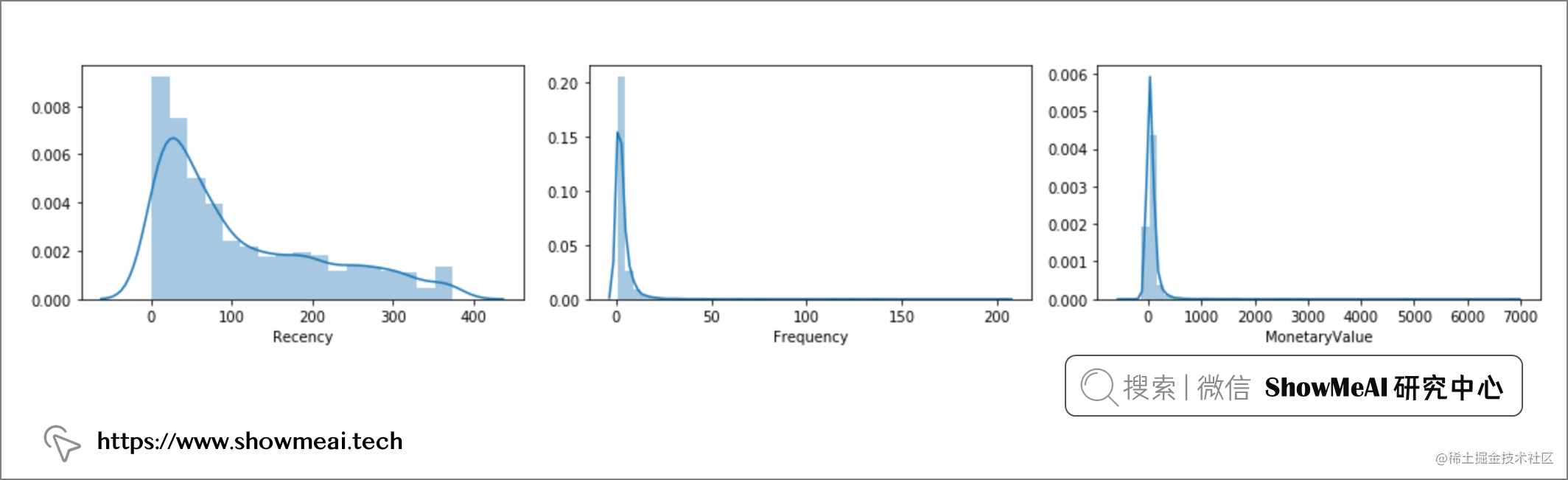• 对数转换
• 平方根变换
• box-cox 变换

``````from scipy import stats
def analyze_skewness(x):
fig, ax = plt.subplots(2, 2, figsize=(5,5))
sns.distplot(customers[x], ax=ax[0,0])
sns.distplot(np.log(customers[x]), ax=ax[0,1])
sns.distplot(np.sqrt(customers[x]), ax=ax[1,0])
sns.distplot(stats.boxcox(customers[x]), ax=ax[1,1])
plt.tight_layout()
plt.show()

print(customers[x].skew().round(2))
print(np.log(customers[x]).skew().round(2))
print(np.sqrt(customers[x]).skew().round(2))
print(pd.Series(stats.boxcox(customers[x])).skew().round(2))
analyze_skewness('Recency')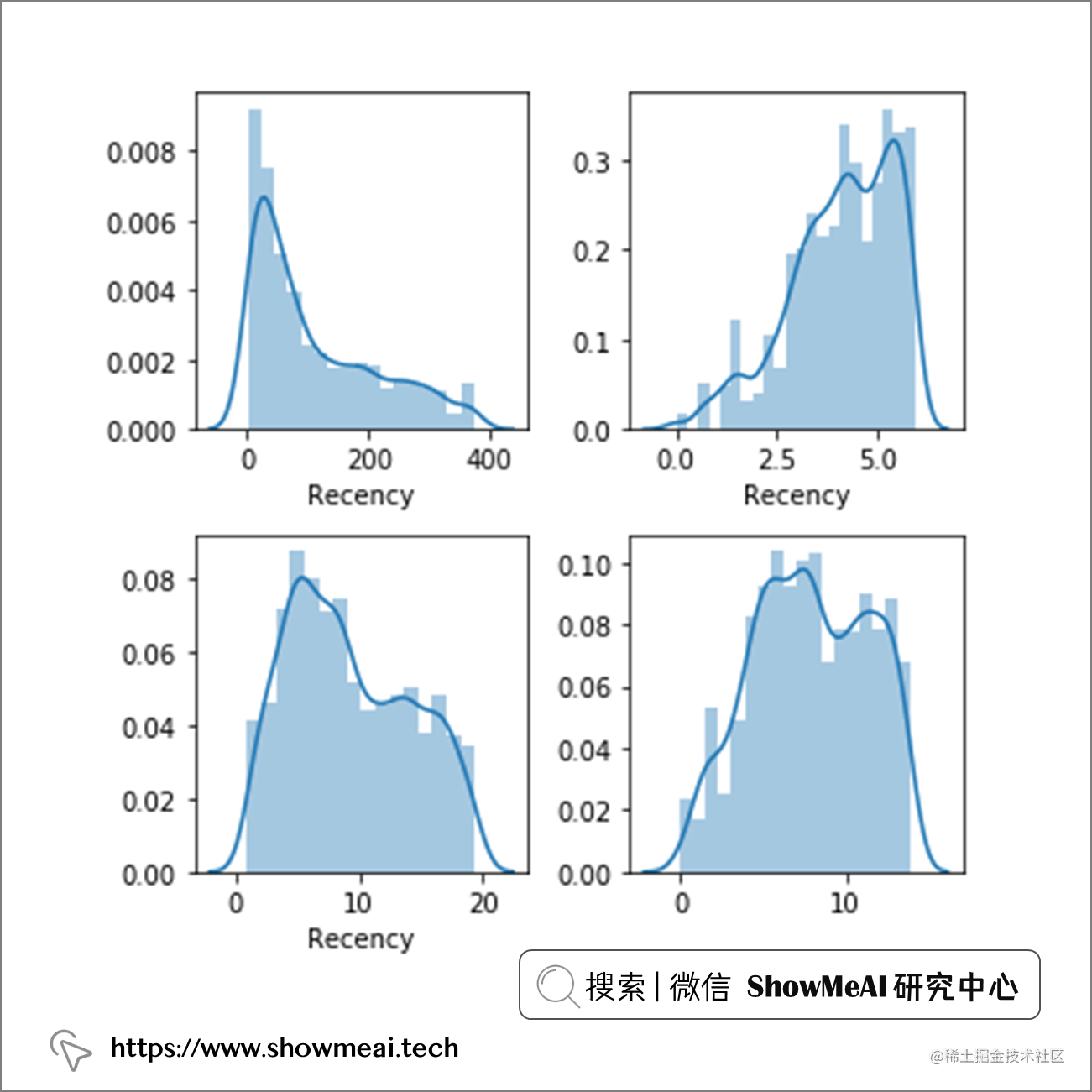``````analyze_skewness('Frequency')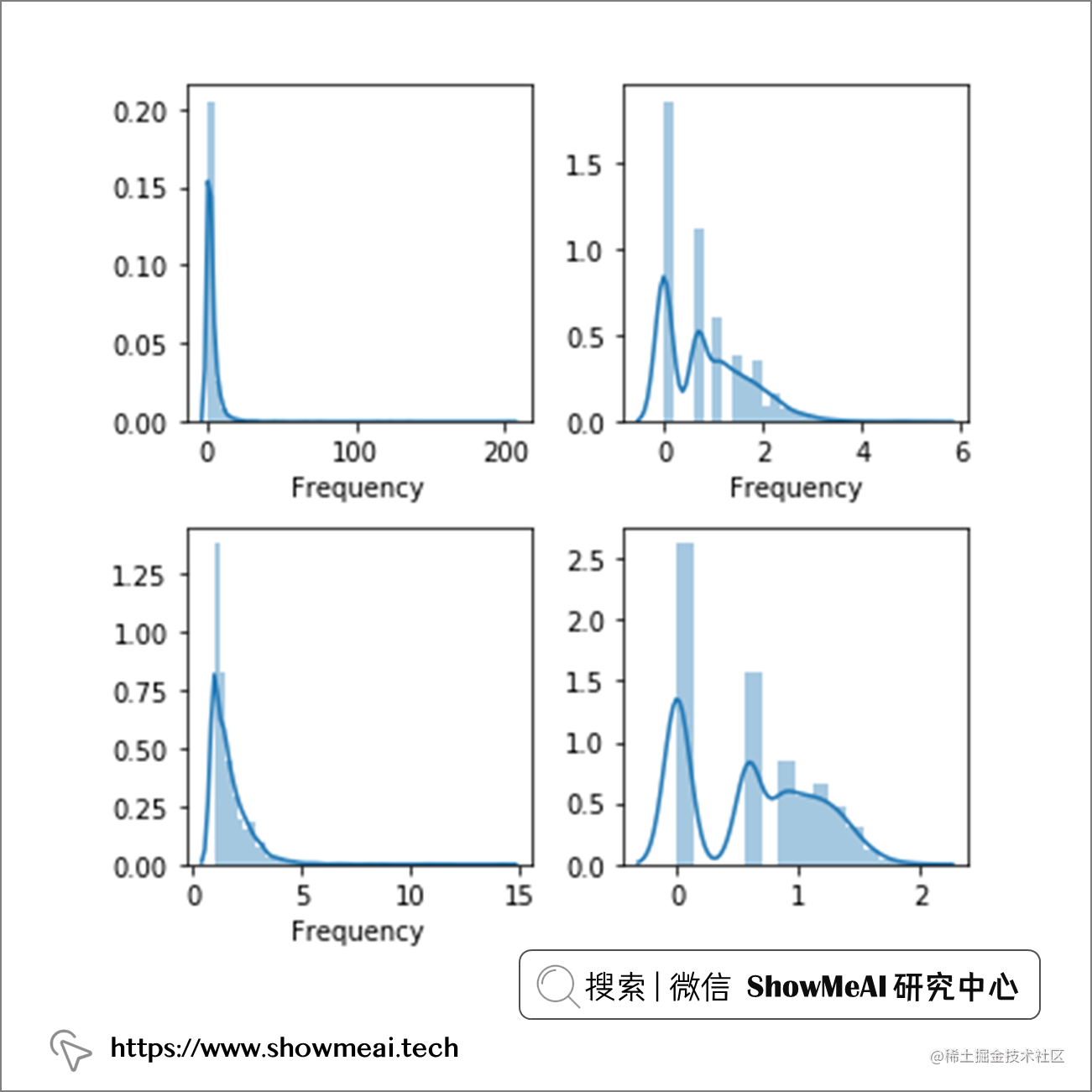``````fig, ax = plt.subplots(1, 2, figsize=(10,3))
sns.distplot(customers['MonetaryValue'], ax=ax)
sns.distplot(np.cbrt(customers['MonetaryValue']), ax=ax)
plt.show()
print(customers['MonetaryValue'].skew().round(2))
print(np.cbrt(customers['MonetaryValue']).skew().round(2))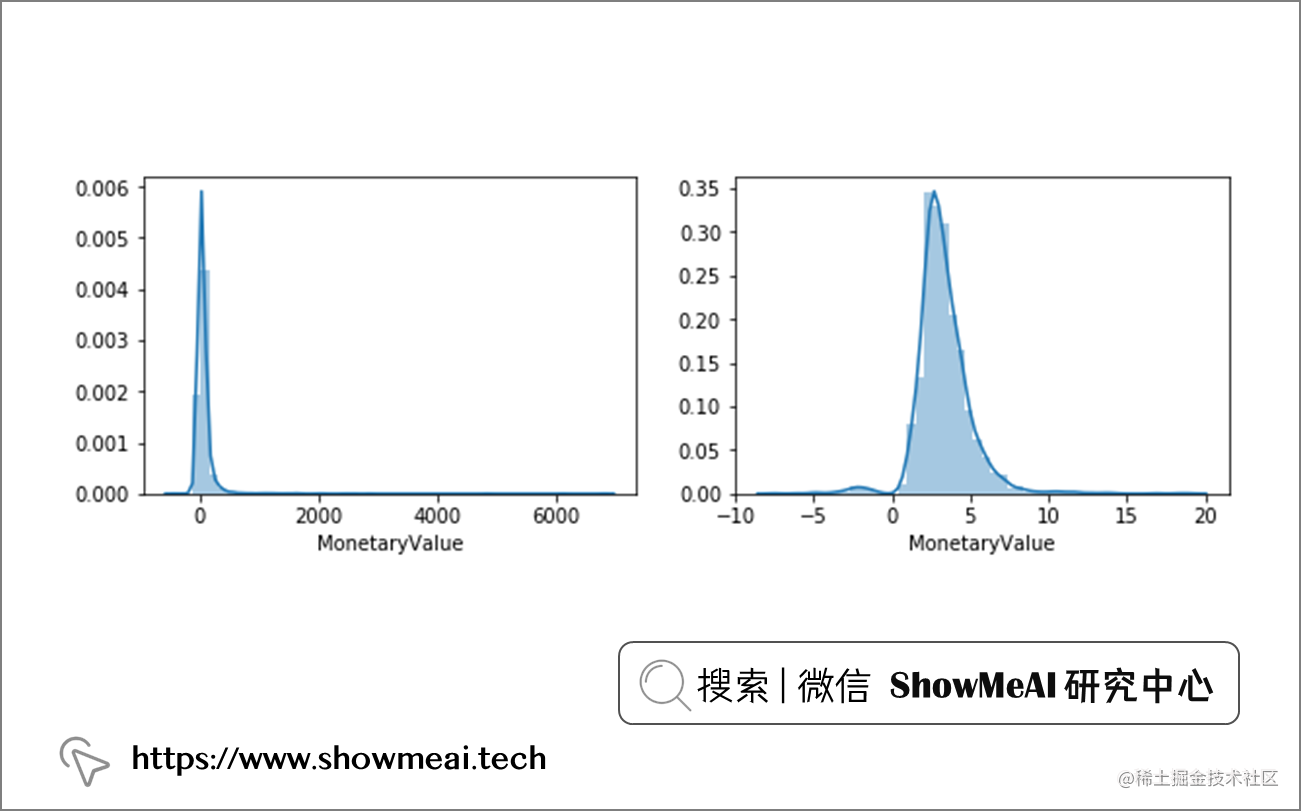``````from scipy import stats
customers_fix = pd.DataFrame()
customers_fix["Recency"] = stats.boxcox(customers['Recency'])
customers_fix["Frequency"] = stats.boxcox(customers['Frequency'])
customers_fix["MonetaryValue"] = pd.Series(np.cbrt(customers['MonetaryValue'])).values
customers_fix.tail()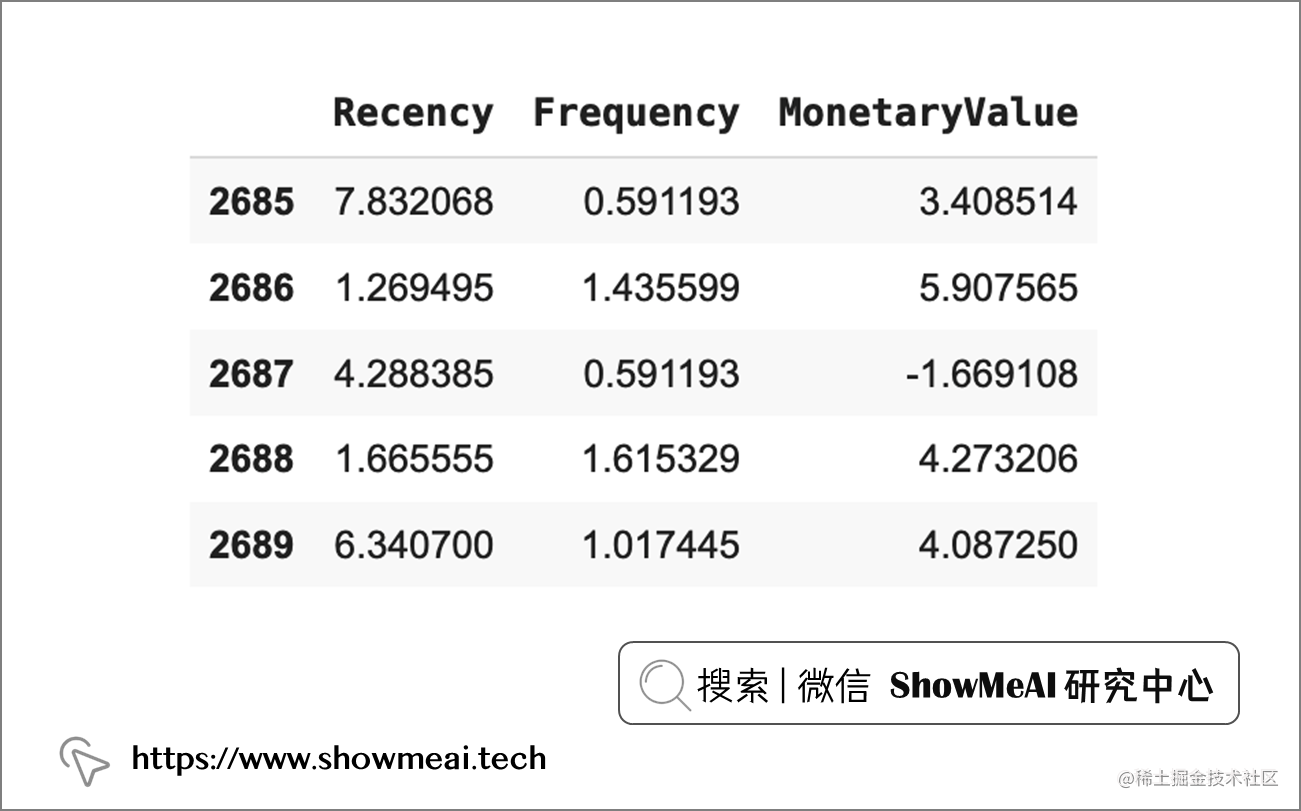``````# 导入库
from sklearn.preprocessing import StandardScaler

# 初始化对象
scaler = StandardScaler()

# 拟合和转换数据
scaler.fit（customers_fix）
customers_normalized = scaler.transform(customers_fix)

# 均值为 0，方差为 1
print(customers_normalized.mean(axis = 0).round(2)) # [0. -0。 0.]
print(customers_normalized.std(axis = 0).round(2)) # [1. 1. 1.]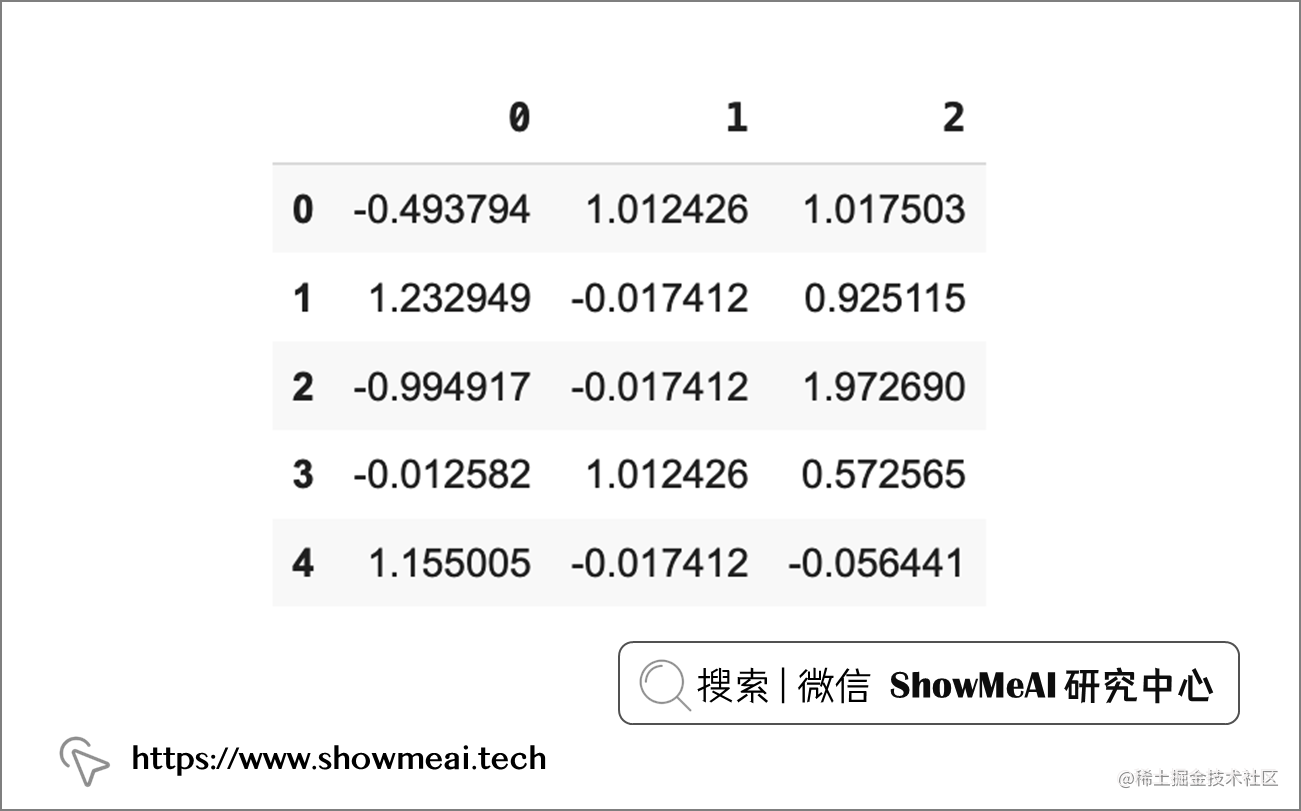# 💡 建模与分群

K-Means 算法是一种无监督学习算法，它通过迭代和聚合来根据数据分布确定数据属于哪个簇。关于 K-Means 的详细知识欢迎大家查看ShowMeAI的教程文章：

``````from sklearn.cluster import KMeans

sse = {}
for k in range(1, 11):
kmeans = KMeans(n_clusters=k, random_state=42)
kmeans.fit(customers_normalized)
sse[k] = kmeans.inertia_

plt.title('The Elbow Method')plt.xlabel('k')
plt.ylabel('SSE')
sns.pointplot(x=list(sse.keys()), y=list(sse.values()))
plt.show()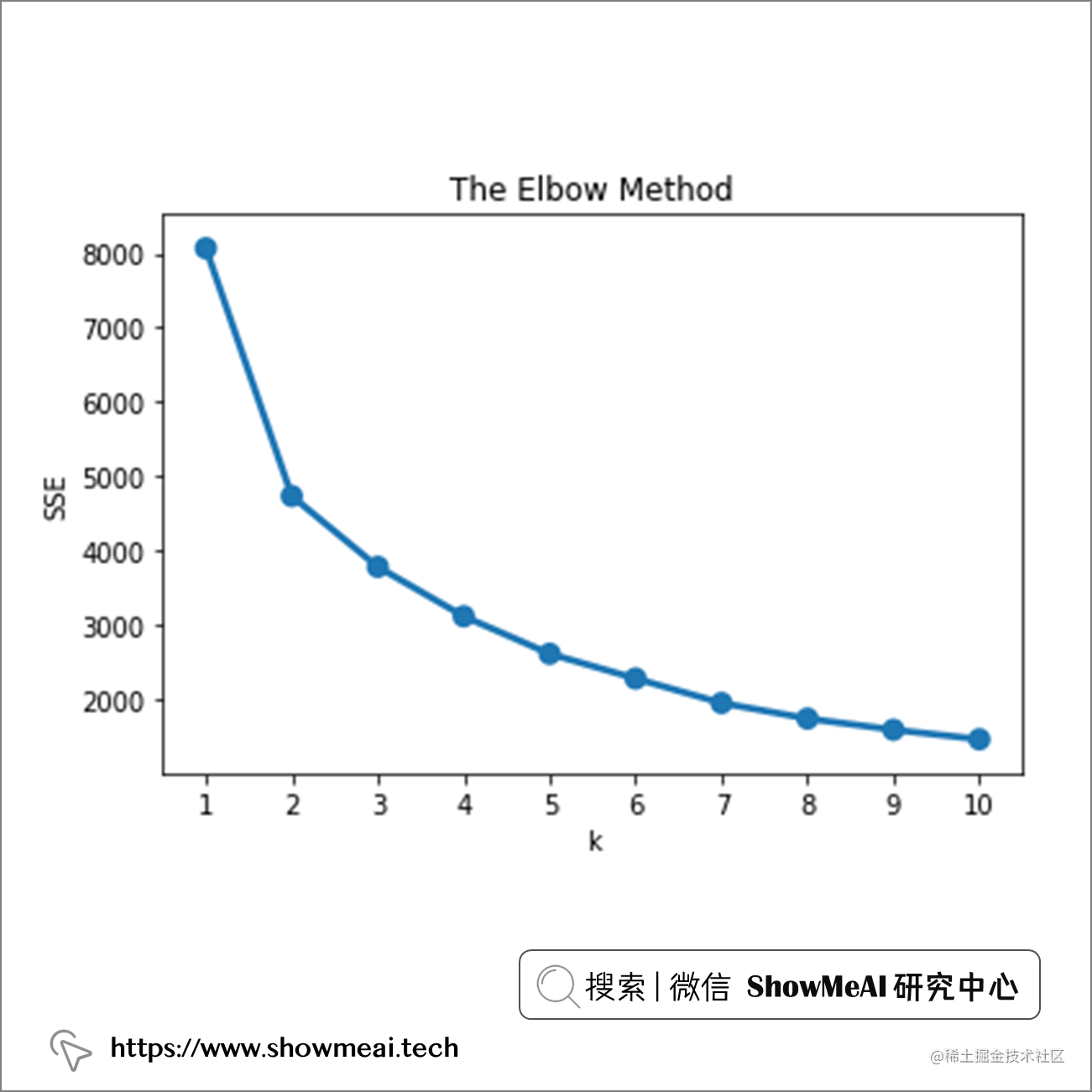``````model = KMeans(n_clusters=3, random_state=42)
model.fit(customers_normalized)
model.labels_.shape

# 💡 模型解释&业务理解

``````customers["Cluster"] = model.labels_
customers.groupby('Cluster').agg({
'Recency':'mean',
'Frequency':'mean',
'MonetaryValue':['mean', 'count']}).round(2)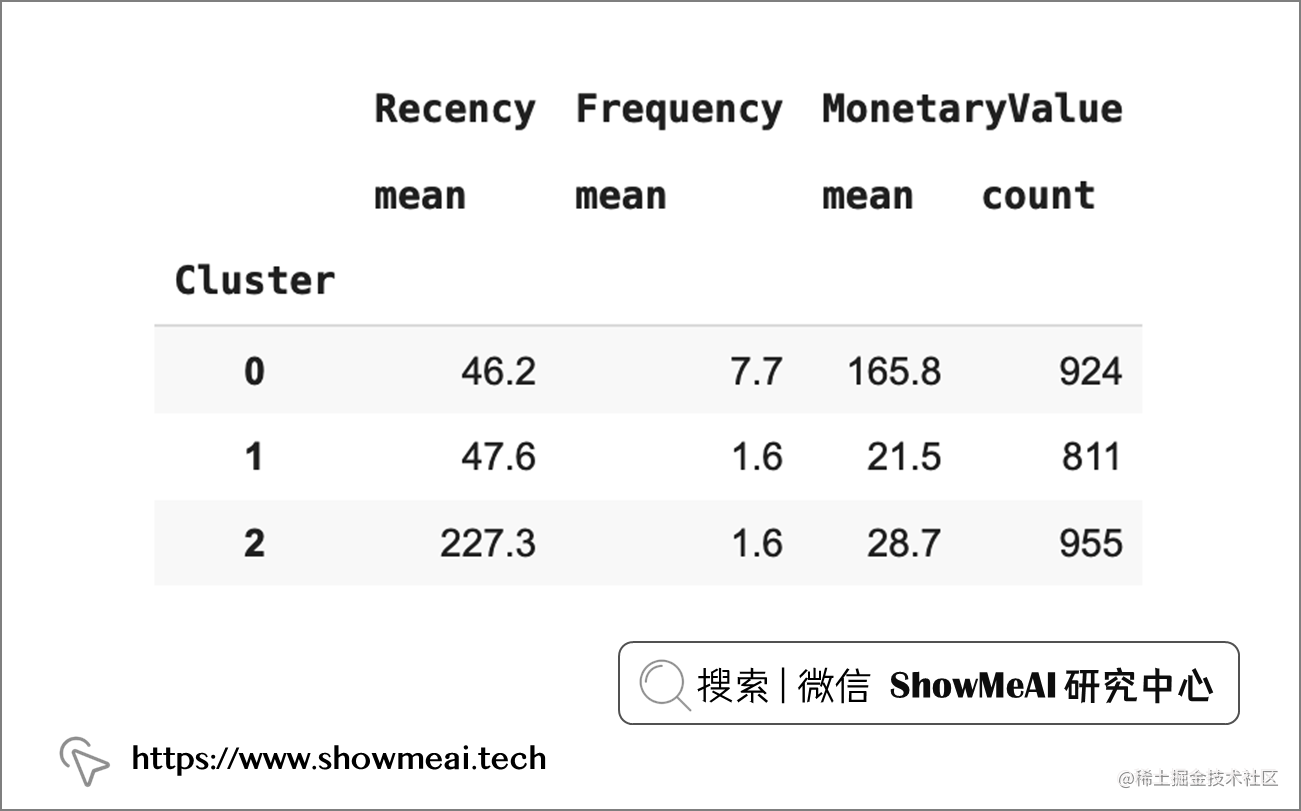• 用户群0：频繁消费，消费数额大，且最近有购买行为。可以视作『忠实客户群』。
• 用户群1： 消费频率较低，消费数额小，但最近有购买行为。可以视作『新客户群』。
• 用户群2：消费频率较低，消费数额小，上一次购买的时间较早。可以视作『流失客户群』。# 参考资料

• 📚 Daqing C., Sai L.S, and Kun G. Data mining for the online retail industry: A case study of RFM model-based customer segmentation using data mining (2012), Journal of Database Marketing and Customer Strategy Management.
• 📚 Millman K. J, Aivazis M. Python for Scientists and Engineers (2011), Computing in Science & Engineering.
• 📚 Radečić D. Top 3 Methods for Handling Skewed Data (2020), Towards Data Science.
• 📚 Elbow Method for optimal value of k in KMeans, Geeks For Geeks.
• 📘 图解数据分析：从入门到精通系列教程www.showmeai.tech/tutorials/3…
• 📘 数据科学工具库速查表 | Pandas 速查表www.showmeai.tech/article-det…
• 📘 数据科学工具库速查表 | Seaborn 速查表www.showmeai.tech/article-det…
• 📘 机器学习实战 | 机器学习特征工程最全解读www.showmeai.tech/article-det…
• 📘 图解机器学习 | 聚类算法详解www.showmeai.tech/article-det…